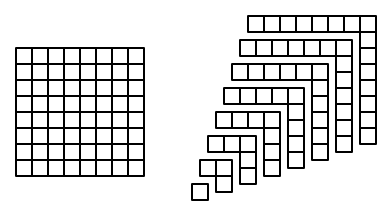$\def\compare{ {\mathrm{compare}} } \def\swap{ {\mathrm{swap}} } \def\sort{ {\mathrm{sort}} } \def\true{ {\mathrm{true}} } \def\false{ {\mathrm{false}} } \def\total{ {\mathrm{total}} }$

Consider the following method that on input a positive integer $$n$$ sums the odd numbers from $$1$$ to $$2 n - 1$$:

1
2
3
4
5
6
Sum(n):
total <- 0
for k = 1 up to n do
total <- total + 2 * k - 1
endfor


Observe that for $$n = 1, 2, 3, 4$$, $$\mathrm{Sum}(n)$$ returns the values $$1, 4, 9, 16$$. In each case, the value returned by $$\mathrm{Sum}(n)$$ is $$n^2$$. Use induction to argue that this formula always holds: for every positive integer $$n$$, the value returned by $$\mathrm{Sum}(n)$$ is $$n^2$$.

### Solution

Consider the for loop in lines 3-5. We claim that for each iteration $$k$$, the value of $$\total$$ at the end of iteration $$k$$ is $$k^2$$. We prove this claim by induction.

Base case. For the base case, $$k = 1$$, note that before the first iteration of the loop, we have $$\total = 0$$ (from line 2). Therefore, in iteration $$k = 1$$, at line line 4 $$\total$$ gets assigned the value $$0 + 2 * (1) - 1 = 1 = k^2$$. Therefore the claim holds for $$k = 1$$.

Induction step. Now suppose the claim holds for some value of $$k$$. That is, after iteration $$k$$, we have $$\total = k^2$$. Consider iteration $$k + 1$$. Then in line 4, $$\total$$ is assigned the value

\begin{align*} \total + 2 (k + 1) - 1 &= k^2 + 2 k + 2 - 1\\ &= k^2 + 2 k + 1\\ &= (k + 1)^2. \end{align*}

Thus, after iteration $$k+1$$, we have $$\total = (k+1)^2$$, so the claim holds.

Since both the base case and inductive step hold, the claim holds for all iterations $$k$$ by induction. Finally, observe that loop terminates after iteration $$n$$, so the value of $$\total$$ returned by $$\mathrm{Sum}(n)$$ is $$n^2$$.

### A Note on the Formula

Given a positive integer $$n$$, we can interpret the value $$n^2$$ geometrically as the area of a square with side length $$n$$. For example, the square figure below (left) has side length 8, and is (evidently) comprised of $$64 = 8^2$$ unit squares.We can decompose the $$8 \times 8$$ square grid into $$8$$ “L”-shapes as on the figure on the right. The “L”s have width and height $$1, 2, 3, \ldots, 8$$, and their areas are $$1, 3, 5,\ldots 15$$. Since the figure on the right is just a partition of the figure on the left, they have the same area. Thus, the figures give a geometric interpretation to the formula

$n^2 = 1 + 3 + 5 + \cdots + 2 n - 1.$